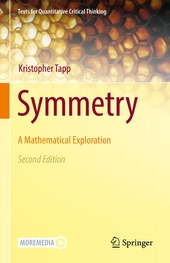# Symmetry

A Mathematical Exploration

Springer (Verlag)
• 2. Auflage
• |
• erscheint ca. am 20. November 2020

• Buch
• |
• Hardcover
• |
• VIII, 336 Seiten
978-3-030-51668-0 (ISBN)

This textbook is perfect for a math course for non-math majors, with the goal of encouraging effective analytical thinking and exposing students to elegant mathematical ideas. It includes many topics commonly found in sampler courses, like Platonic solids, Euler's formula, irrational numbers, countable sets, permutations, and a proof of the Pythagorean Theorem. All of these topics serve a single compelling goal: understanding the mathematical patterns underlying the symmetry that we observe in the physical world around us.

The exposition is engaging, precise and rigorous. The theorems are visually motivated with intuitive proofs appropriate for the intended audience. Students from all majors will enjoy the many beautiful topics herein, and will come to better appreciate the powerful cumulative nature of mathematics as these topics are woven together into a single fascinating story about the ways in which objects can be symmetric.

 Reihe: Auflage: 2nd ed. 2020 Sprache: Englisch Verlagsort: Cham | Schweiz Verlagsgruppe: Springer International Publishing Zielgruppe: Für Beruf und Forschung Editions-Typ: Überarbeitete Ausgabe Illustrationen: 11 s/w Abbildungen, 154 farbige Tabellen, 289 farbige Abbildungen | 154 Tables, color; 289 Illustrations, color; 11 Illustrations, black and white; VIII, 336 p. 300 illus., 289 illus. in color. Maße: Höhe: 23.5 cm | Breite: 15.5 cm Schlagworte: ISBN-13: 978-3-030-51668-0 (9783030516680) DOI: 10.1007/978-3-030-51669-7weitere Ausgaben werden ermittelt

Kristopher Tapp is Professor of Mathematics at Saint Joseph's University in Philadelphia. The recipient of numerous teaching awards, he is particularly interested in communicating ideas from mathematics to students in other fields. His research extends from differential geometry to the mathematical study of gerrymandering. Previous books include Differential Geometry of Curves and Surfaces, which appears in Undergraduate Texts in Mathematics.

Preface.- 1. Introduction to Symmetry.- 2. The Algebra of Symmetry.- 3. The Classification Theorems.- 4. Isomorphic Groups.- 5. Subgroups & Product Groups.- 6. Permutation Groups.- 7. Symmetries of 3D Objects.- 8. The Five Platonic Solids.- 9. Symmetry and Optimization.- 10. What is a Number?.- 11. Excursions in Numbers.- 12. Rigid Motions as Functions.- 13. Rigid Motions as Matrices.- Image Credits.- Index.
This textbook invites readers to explore the properties of objects that we effortlessly recognize as symmetrical. From the human body, to elaborate artworks and abstract networks, symmetry is ubiquitous in the world around us; it is essential to function and beauty across the built and natural world. By developing mathematical language to describe symmetry, this textbook equips readers to explore symmetries with precision, illuminating commonalities across art and science.

Beginning with an exploration of intuitive notions of symmetry, the author introduces the algebraic structure of groups by examining rotations and reflections. Topics that follow include the classification of two-dimensional patterns, the Platonic solids, and a study of real and prime numbers. This second edition features numerous new examples and exercises, "Elements of Mathematics" sections that more closely examine mathematical tools, and optional content that offers opportunities for extension. Figures are woven into the presentation throughout, with many illustrations now offered in motion as integrated video content.

Symmetry suits a wide audience, demanding no mathematical training past high school basics, yet stretching the imagination of math and non-math majors alike. Its engaging yet rigorous style offers a window into mathematicians' ways of thinking. By developing mathematical reasoning skills in a spatial context, this text is ideal for a course that meets quantitative requirements for non-STEM majors. Instructors will appreciate the author's comprehensive online resources, which include a full set of animated lecture slides; all readers will delight in watching the book's content come alive using the multimedia app.
Schweitzer Klassifikation
• Mathematik
•Algebra
•Abstrakte Algebra
• Mathematik
•Geometrie
•Euklidische Geometrie
• Mathematik
•Gruppentheorie
Thema Klassifikation
Newbooks Subjects & Qualifier
DNB DDC Sachgruppen
Dewey Decimal Classfication (DDC)
• Science
•Mathematics
•Algebra
• Science
•Mathematics
•Geometry
• Science
•Mathematics
•Mathematics
• Science
•Mathematics
•Probabilities & applied mathematics
BIC Classifikation
• Mathematics & science
•Mathematics
•Applied mathematics
• Mathematics & science
•Mathematics
•Geometry
• Mathematics & science
•Mathematics
•Groups & group theory
BISAC Classifikation
• Mathematics
•Algebra / General
•Abstract
• Mathematics
•Applied
Warengruppensystematik 2.0

Noch nicht erschienen

 53,49 € inkl. 5% MwSt.